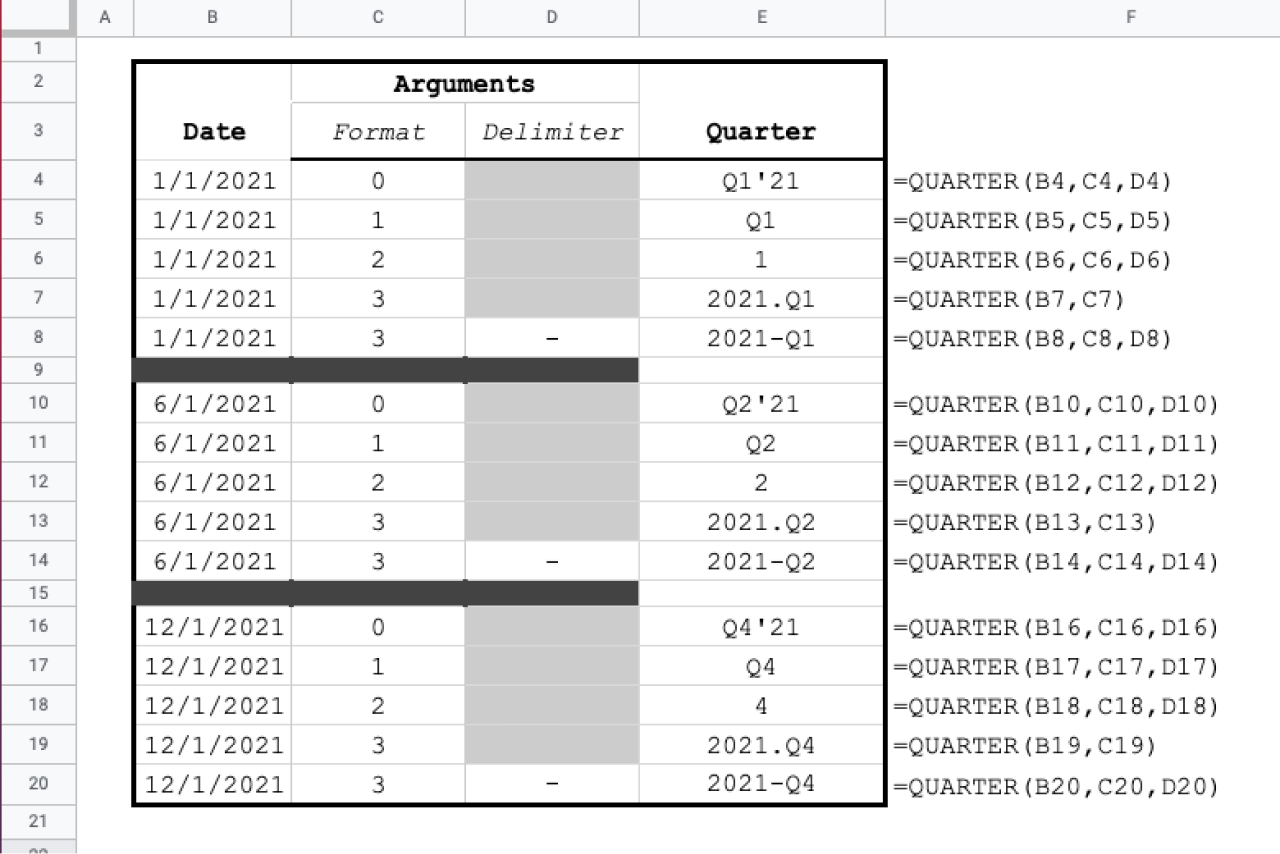# Transform dates to financial quarter in Google Sheets

Jul 7

The QUARTER function is an essential tool for pivoting and grouping data by quarter.

### Function Signature

`=QUARTER(input, format, delimiter)`

### Argument Definitions

[range of dates] input; required - date or range of dates to convert. If string or number is passed, the function attempts to convert to a date.

[number] format - how to generate combinations

• 0 - Q1'YY - Q4'YY [default]
• 1 - Q1 - Q4
• 2 - Number of quarter: 1, 2, 3 or 4
• 3 - yyyy.Q1 - yyyy.Q4

[string] delimiter - also known as the separator for items in a given combination

## Use the Cheat

Click the Code Box to Copy

Cheat copied to clipboard ✂️👍
``` /** * Create key for financial quarter for a date. * * @param {Date} input - input date * @param {number} format - quarter key type * - 0 - Q1'YY - Q4'YY [default] * - 1 - Q1 - Q4 * - 2 - a number 1 - 4 * - 3 - yyyy.Q1 - yyyy.Q4 * * @param {string} delimiter - separator for return format 3 only * @param {boolean} add_header - true by default, for array input the function returns a header "Quarter" * @return financial quarter of date based on format parameter. * * @customfunction */ function QUARTER(input, format = 0, delimiter = '.' ) { const is_array = input instanceof Array; let ret = [[]]; if (input instanceof Array) { const is_matrix = input.length > 1; for (let i=0; i < input.length; i++) { if (is_matrix) { for (let j=0; j < input[i].length; j++) { if (!ret[i]) { ret[i] = []; } ret[i].push(single_quarter_(input[i][j], format, delimiter)); } } else { if (!ret[i]) { ret[i] = []; } ret[i].push(single_quarter_(input[i], format, delimiter)); } } } else { ret = [single_quarter_(input, format, delimiter)]; } return ret; }; function single_quarter_(input, format = 0, delimiter = '.') { if (!(input instanceof Date)) { input = new Date(input); } const q = input.getQuarter(); const year = input.getFullYear(); switch(format) { case 1: return "Q" + q; case 2: return q; case 3: return year.toString() + delimiter + "Q" + q; }; // default case let year_str = year.toString(); if (year < 1000) { return "The year is prior to 1000 AD. This looks like an error. If not, email us at support@lassie.ai to tell us what's up." } return "Q" + q + "'" + year_str.substring(year_str.length-2, year_str.length); } Date.prototype.getQuarter = function() { const q = (this.getMonth() / 3) + 1; return Math.floor(q); }; ```Cyrus Radfar